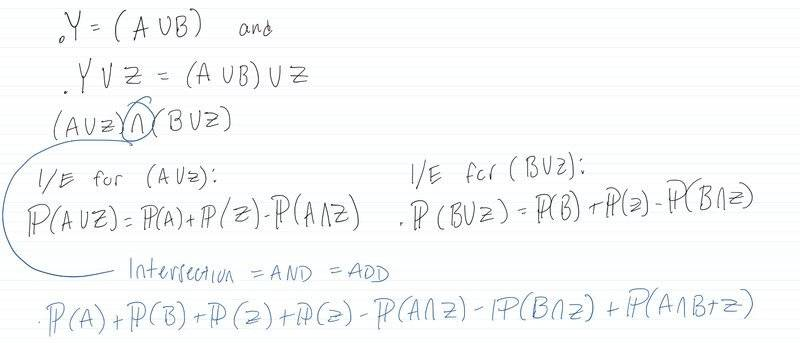# Inclusion/Exclusion Principle for 3 events

guyvsdcsniper
Homework Statement:
Prove the I/E formula for 3 events by 1st applying
I/E to (A∪B) ∪Z. In other words, consider (A∪B) as a
single event "Y" and then apply I/E between "Y" and Z.
Relevant Equations:
P(A∪B)=P(A)+P(B)−P(A∩B)

P(A∪B∪C)=P(A)+P(B)+P(C)−
−P(A∩B)−P(A∩C)−P(B∩C)+P(A∩B∩C)
Below is my attempt at the problem. I used the distributive law and applied to what was given, (A∪B)∪Z which equates to (A∪Z)∩(B∪Z). I then applied the 2 set I/E formula to each union. Since there is an intersection between these two sets I added them.
But I end up with 2 P(Z) which doesn't seem right.

I feel like I am got the distributive property part down but definitely getting something wrong past that.fishturtle1
I have a little trouble following it, but it looks like you've made a mistake in distribution e.g., ##(A \cup B) \cup Z \neq (A \cup Z) \cap (B \cup Z)## in general.

We have ##P(Y \cup Z) = P(Y) + P(Z) - P(Y \cap Z)##. We can then apply I/E to ##P(Y)## (i'll leave that to you). We can also use distribution (as you suggested) to get
$$Y \cap Z = (A \cup B) \cap Z = (A \cap Z) \cup (B \cap Z)$$

And now we can use I/E on ##P((A \cap Z) \cup (B \cap Z))## (what are the two events?).

Putting it all together should give us the identity!

•PeroK
Prof B
Homework Statement:: Prove the I/E formula for 3 events by 1st applying
I/E to (A∪B) ∪Z. In other words, consider (A∪B) as a
single event "Y" and then apply I/E between "Y" and Z.
Relevant Equations:: P(A∪B)=P(A)+P(B)−P(A∩B)

P(A∪B∪C)=P(A)+P(B)+P(C)−
−P(A∩B)−P(A∩C)−P(B∩C)+P(A∩B∩C)

Below is my attempt at the problem. I used the distributive law and applied to what was given, (A∪B)∪Z which equates to (A∪Z)∩(B∪Z). I then applied the 2 set I/E formula to each union. Since there is an intersection between these two sets I added them.
But I end up with 2 P(Z) which doesn't seem right.

I feel like I am got the distributive property part down but definitely getting something wrong past that.

View attachment 301811
The instructions say to apply I/E to Y U Z. You didn't do that.

As for the rest, identities have a right side and a left side. You have to memorize both. You can't just memorize the right side and then write down whatever you feel like for the left side.

Regardless, there is no need to pick your favorite law from your list of identities. The instructions are really clear about what to do first.